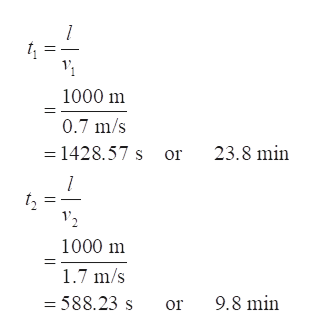# A River has a steady speed of 0.500 m/s. A student swims upstream a distance of 1.00 km and swims back to the startingpoint.(a) If the student can swim at a speed of 1.20 m/s in still water, how long does the trip take? (b) How much time is required in still water for the same length swim?(c) Intuitively, why does the swim take longer when there is a current?

Question
347 views

A River has a steady speed of 0.500 m/s. A student swims upstream a distance of 1.00 km and swims back to the starting
point.

(a) If the student can swim at a speed of 1.20 m/s in still water, how long does the trip take? (b) How much time is required in still water for the same length swim?

(c) Intuitively, why does the swim take longer when there is a current?

check_circle

Step 1

Consider the motion of the student when the river has some speed. Let vr be the speed of the river, vs be the speed of the student, v1 be the relative speed of student with respect to the river travelling upstream, and v2 be the relative speed of the student with respect to the river travelling downstream. Let l be the distance travelled by the student.

Write the given information in the question.

Step 2

Write the expression for the relative velocity of student with respect to the river travelling upstream and downstream.

Step 3

Let t1 be the time taken for travelling upstream, and t2 be the time taken to tra...help_outlineImage Transcriptionclose1000 m 0.7 m/s 23.8 min 1428.57 s or 1000 m 1.7 m/s = 588.23 s 9.8 min or fullscreen

### Want to see the full answer?

See Solution

#### Want to see this answer and more?

Solutions are written by subject experts who are available 24/7. Questions are typically answered within 1 hour.*

See Solution
*Response times may vary by subject and question.
Tagged in